## Comparison of Conductor Material in Underground System:

In an Conductor Material in Underground System using multi-core belted type cables, the chief stress on the insulation is usually between conductors. Under such situations, comparison is made on the basis of maximum voltage between conductors.

• The same power (P watts) is transmitted by each system.
• The distance (l metres) over which power is transmitted remains the same.
• The line losses (W watts) are the same in each case.
• The maximum voltage between conductors (Vm) is the same in each case.

### 1. Two Wire DC System:

If Vm denotes the maximum potential difference between the conductors, it will also be the working voltage in this case.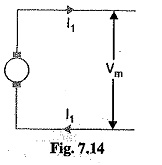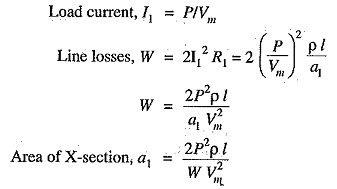Volume of Conductor Material in Underground System required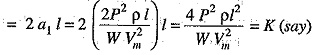This volume will be taken as the basic quantity and comparison shall be made for other systems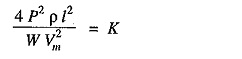###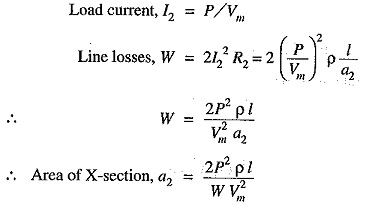Volume of conductor material required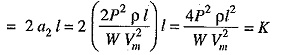Hence, the volume of Conductor Material in Underground System required in this system is the same as that for Two Wire DC System.

### 3. Three Wire DC System:

The maximum voltage between the outers is Vm. Assuming balanced load, the current through the neutral wire will be zero.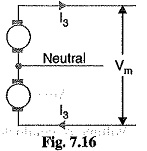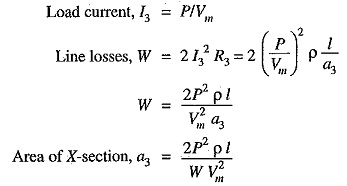Assuming the area of X-section of neutral wire to be half of that of either outers,

Volume of conductor material required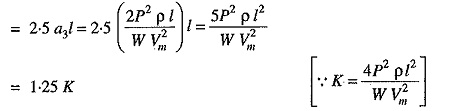Hence, the volume of Conductor Material in Underground System required in the system is 1.25 times that required for Two Wire DC System.

### 4. Single Phase 2 Wire AC System:

As the maximum voltage between conductors is Vm therefore, r.m.s. value of voltage is Vm/√2. Assuming the p.f. of the load to be cos Φ,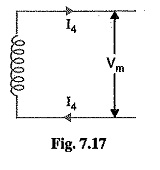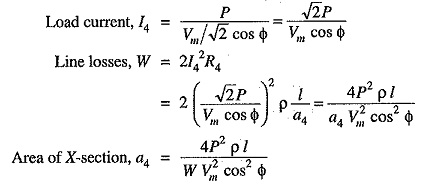Volume of conductor material required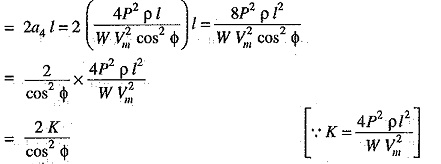Hence the volume of Conductor Material in Underground System required for this system is 2/cos2Φ times that required in a 2-wire d.c. system.

### 5. Single Phase 2 Wire System with Mid Point Earthed:

The maximum value of voltage between the outers is Vm. Therefore, the r.m.s. value of voltage is Vm/√2. If the p.f.of the load is cos Φ,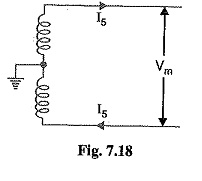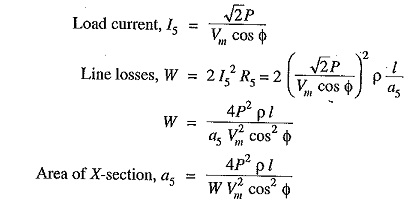Volume of conductor material required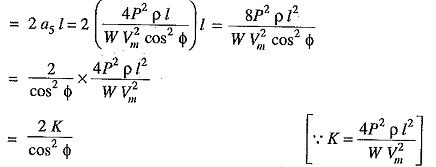Hence, the volume of Conductor Material in Underground System required in this system is 2/cos2Φ times that required in 2-wire d.c. system.

### 6. Single Phase 3 Wire System:

If the load is considered balanced, the system reduces to a single phase 2-wire except that a neutral wire is provided in addition. Assuming the area of X-section of the neutral wire to be half of either of the outers,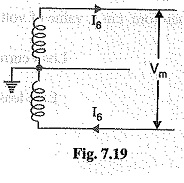Volume of conductor material required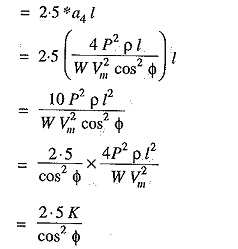Hence, the volume of Conductor Material in Underground System required in this system is 2.5/cos2 Φ times that required in 2 wire DC system.

### 7. Two Phase 4 Wire System:

This system can be considered as two independent single phase systems, each transmitting one-half of the total power. It is clear form Fig. 7.20 that voltage across outers (AB or CD) is twice that of single phase 2-wire (refer back to Fig. 7.17). Therefore, current (I7) in each conductor will be half that in Single Phase 2 Wire System. Consequently, area of X-section of each conductor is also half but as there are four wires, so volume of conductor material used is the same as in a single phase, 2-wire system i.e.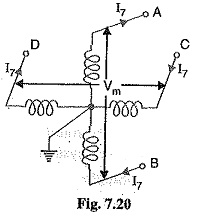Volume of conductor material required.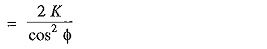Hence, volume of conductor material required in this system is 2/cos2Φ times that required in 2-wire d.c. system.

### 8. Two Phase 3 Wire System:

Fig. 7.21 shows Two Phase 3 Wire System. Let us suppose that maximum voltage between the outers is Vm. Then maximum voltage between either outer and neutral wire is Vm/√2.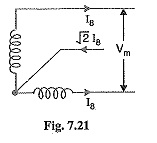R.M.S. voltage between outer and neutral wire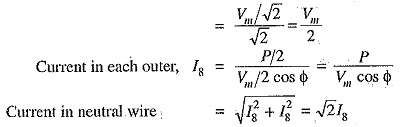Assuming the current density to be constant, the area of X-section of neutral wire will be √2 times that of either of the outers.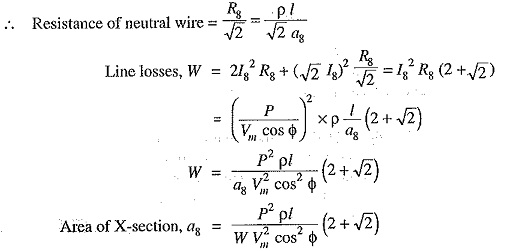Volume of conductor material required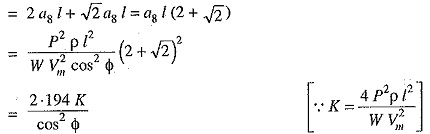Hence, the volume of conductor material required in this system is 2.914/cos2Φ times that required in 2-wire d.c. system.

### 9. 3 Phase 3 Wire system:

Suppose that the maximum value of voltage between the conduc­tors is Vm. Then maximum voltage between each phase and neutral is Vm/√3. Therefore, r.m.s. value of voltage per phase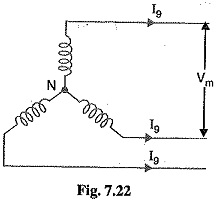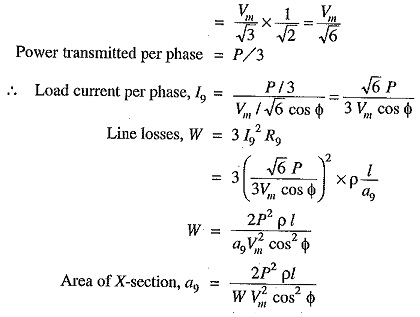Volume of conductor material required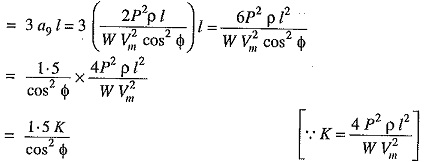Hence, the volume of Conductor Material in Underground System required in this system is 1.5/cos2Φ times that required in 2-wire d.c. system.

### 10. 3-Phase 4-Wire System:

Fig. 7.23 shows the 3-Phase 4-Wire System. If the loads are balanced, then neutral wire carries no current. Consequently, the system reduces to a 3-phase, 3-wire system except that there is additional neutral wire. Assuming the area of X-section of the neutral wire to be half that of line conductor,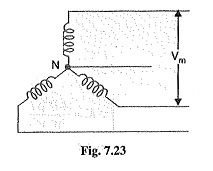Volume of conductor material requiredHence the volume of Conductor Material in Underground System required in this system is 1.75/cos2Φ times that required in 2-wire d.c. system.

Scroll to Top